Chordal and linear structures In the generation of triads and scales, 3 diagrams were placed side by side -1. in the center, the original series of fifths in the diatonic Window (7 notes)     which acts as generator for both structures (chordal and linear);2. on the left, where the FRAMEs of fifths are “divided” by the MEDIANs,     we find the chordal structure of triads          (where all the notes are heard at the same time); and3. on the right, the double circle of fifths, where the fifths are “multiplied” by 2,     we find the linear structure of the scale,          (where notes are heard one after the other). With Jordan Triangles, it will be possible1. to combine both structures (chordal and linear) in one diagram,2. to extend the possibilities of linear structure “multiplication” from 2 fifths to 3,     (possibly more) and3. to observe the “birth” (borrowing) of the MOTRIX (sixth or seventh) more clearly. Definition of the Jordan Triangle Orbits 0 and 1Starting from the central note D, acting as the COMMON TONE, Orbit 0, and generating, with the interval of the perfect fifth, the notes G and A, produces the PROPER TONEs, Orbit 1, of the 2 FRAMEs of the swing D.In the normal disposition of the Window,     the 3 notes of this swing are placed vertically,          in a column. However, if the 2 extremities of the swing          (G and A, Orbit 1),     are placed to one side,     they may be joined by a vertical green line,          producing a “Jordan Triangle”. Chordal and linear structural relationshipsBoth of these relationships are present in the triangle.1 - The blue lines D-G and D-A represent     (a) not only the chordal relationship of the 2 FRAMEs of the swing D          (the pivotal COMMON TONE gives the swing its name),     (b) but also the linear Voice-leadings 0-1 and 1-0 (the Fundamental Bass).2 - The green line G-A represents     (a) not only the chordal relationship between the COMMON TONE and the MOTRIX,     (b) but also the basic Voice-leading 1-1          (between the two peripheral PROPER TONEs).Assemblage of Jordan Triangles Orbits 2 and 3The 5 triangles of the Window (one for each swing) can be superimposed in a column     by zig-zagging the notes of the series of fifths (F, C, G, D, A, E, B).The linear structure of the scale is found in the vertical green lines on each side     (C, D, E, on the right and F, G, A, B, on the left).The triangles are separated from each other to clearly differentiate Orbit 0) and Orbit 1).The same FRAME is indicated twice (with 2 parallel blue lines)     specifying the direction of the chord (the swing to which it belongs)          with Orbit 0 and Orbit 1 indicated accordingly. Insertion of MEDIANS     in the middle of each (double) blue lineThey correspond to the notes of the Window     but have their own specific tuning (+/-),     being generated by the natural major third (5/4).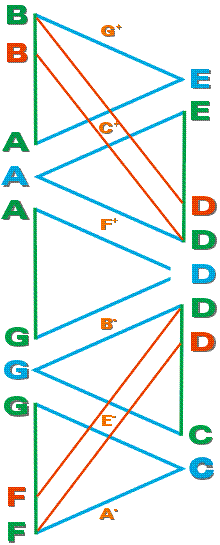The Jordan DiamondsAny 2 adjacent triangles form a diamond,     composed of 2 adjacent swings,     with the common chord between them.We will concentrate on the diamonds at the ends.     (The central swing D,          which unites the major and minor sections,     is, most of the time, only a one-way passage.)The MOTRIX DiagonalsLet us start by examining the 2 red diagonals,     between F and D, in the bottom of the Window.1. The note F is Orbit 1 of the FRAME CF and     the note D is Orbit 1 of the FRAME GD.2. These diagonals run past the note E-,     Orbit 2of the FRAMEs GC and CG.3. These diagonals indicate the linear structures     F E- D and D E- F,          common to both swing G and swing C.4. (a) in the swing G, F-E--D represents          the Orbit line 3-2-1, and     (b) in the swing C, D-E--F represents          the Orbit line 3-2-1.5. The Orbit 3 F of the FRAME GD     is borrowed from the Orbit 1 F of the FRAME CF.The Orbit 3 D of the FRAME CF     is borrowed from the Orbit 1 D of the FRAME GD.One could even say that the Orbit 3 F          is borrowed from the substitution 1(3)     and that the Orbit 3 D          is also borrowed from the substitution 1(3).6. There seems to be a strong symbiosis between the two MOTRIX)s     which resolve to the MEDIAN E- of the TONIC chords GC or CG.7. The MOTRIX, in these F E- D, D E- F diagonals,          seems to have strong linear heritage,     but one must not neglect the chordal relationship of 2 fifths (9/8)          between F and G (on the chord of G7) and          between D and C on the chord of F6.Note that the chordal relationship is between Orbit 0 and Orbit 3     and that the linear relationship is between Orbit 1 and Orbit 1. Where do MOTRICES come from?Each chord surrounding the TONIC (DOMINANT and COUNTER)     borrows its MOTRIX from the substitution of the MOTRIX 1(3) of the other chord.This becomes all the more evident when chromaticism is involved (like the D#--).The same procedure of MOTRIX borrowing will apply elsewhere in the Window     not only in the D-B diagonals, for the MOTRIX of Dm6 and Em7     but also in the C-A diagonals, for the MOTRIX of C6 and Dm7, and     in the G-E diagonals, for the MOTRIX of G6 and Am7.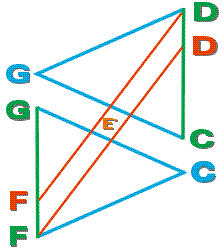1. Comparing the triangle and the diamond(a) In the triangle, we have the primary notes -     one Orbit 0 which is pivotal and     two Orbit 1s which are peripheral.(b) In the diamond, we have the secondary notes -     one Orbit 2, in the center, which is generated and     two Orbit 3s, at the ends, which are borrowed. 2. Comparing the 2 sets of diagonalsIn the F-C-D-G diamond there are     the C E- G, G E- C diagonals which are chordal, and     the F E- D, D E- F diagonals which are linear.Orbit 2(E-) belongs to the 4 diagonals,     with both linear and chordal structure. It is interesting to observe Orbit 2 (E-) surrounded by a “court” of four Orbits 1 -one of which (D or F) will be borrowed to become Orbit 3,and another (G or C) will be chosen to be Orbit 0,depending on the swing (C or G) involved at that moment. The periphery of the Jordan DiamondThis is all the more evident when we examine the complete periphery of the diamond,     with the MEDIANS added, B- in the FRAME GD and A- in the FRAME CF     (see the following illustration in The Four Metamorphoses).(a) On the upper left side, delimited by the F E- D MOTRIX diagonal,     we have the complete DOMINANT chord, D, B-, G, F. (G7).(b) On the lower right side, delimited by the D E- F MOTRIX diagonal,     we have the complete COUNTER chord, F, A-, C, D, (F6).The orbits are inversed in perfect symmetry with     the TONIC triad, on the short diagonals, between its 2 neighboring tetrads. The Four MetamorphosesThis seems to be the best way we have yet found          to illustrate Metamorphosis 3, (G7 / F6) -     Orbit 1and Orbit 3 (D, F/ F, D) exchange notes,     Orbit 2of each chord (B- / A-) acts as a          non-chordal tone of Orbit 0 of the other chord (C / G). The linear resolutions of these non-chordal tones     could be clearly perceived in MOTRIX diagonals          which do not appear in this diagram -     (a) B- to C in the diagonal; A B- C, and     (b) A- to G in the diagonal; Bb A- G. Metamorphosis 3 is the most “compact” of the four,     occupying only 2 Jordan triangles (1 diamond)          because both tetrads use the same diagonal.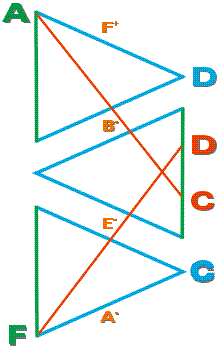Metamorphosis 4 (F6/ Dm7)     is not as compact as Metamorphosis 3,          occupying 3 Jordan triangles. Its chords use 2 sets of MOTRIX diagonals -     F E- D / D E- F, of which we show only          D E- F for the chord of F6 (F, A-, C, D) and     A B- C / C B- A , of which we show only          C B- A for the chord of Dm7 (A, F+, D, C).Orbits 0 and 3 (C, D / D, C) exchange notes,Orbits 1 and 2 (F, A- / A, F+) also exchange notes,     but change pitch in the process (F / F+, A- / A).It is the “spread” over 3 triangles     that is responsible for this change of pitch,          a comma higher in the minor section of the Window.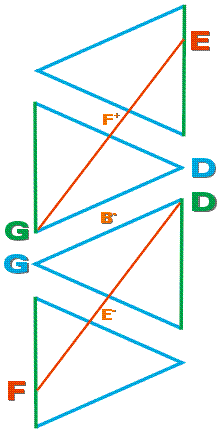Metamorphosis 1 (G6 / G7) is even more spread than     Metamorphosis 4, occupying 4 Jordan triangles. Its chords use 2 sets of MOTRIX diagonals -     G F+ E / E F+ G, of which we show only          E F+ G for the chord of G6 (G, B-, D, E) and     D E- F / F E- D , of which we show only          F E- D for the chord of G7 (D, B-, G, F).As we are dealing with the same triad, G (major),     Orbits 0 and 1 (D, G / G, D)          will evidently exchange notes of the FRAME.Being the MEDIAN of the same triad, G (major),     Orbit 2(B-) evidently remains in the same voice.Orbit 3remains in the same voice but changes note (E / F),     causing a considerable spread for the same triad.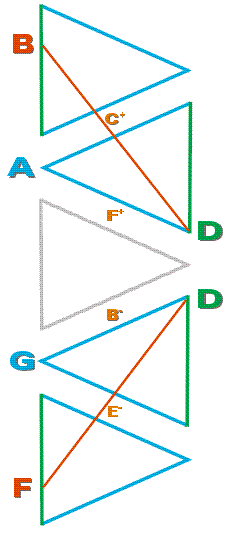Metamorphosis 2 (G7 / Dm6)     is even more spread than Metamorphosis 1,          occupying 5 Jordan triangles (the entire Window). Its chords use 2 sets of MOTRIX diagonals -     D E- F / F E- D , of which we show only          F E- D for the chord of G7 (D, B-, G, F), and     D C+ B / B C+ D, of which we show only          B C+ D for the chord of Dm6 (D, F+, A, B)Orbit 1 (D) belongs to both chords,     and remains in the same voice.Orbits 2 and 3 (B-, F / F+, B)     exchange notes but change pitch (B- / B, F / F+).     Once again, it is this “spread” over 5 triangles          that is responsible for this change of pitch,               a comma higher in the minor section of the Window.Orbit 0 remains in the same voice but changes note (G / A).     The Orbit 0 of each chord acts as a          non-chordal tone of Orbit 0 of the other chord.     The linear relationship of these non-chordal tones          could be clearly perceived in their Orbit 1 relationship (G-A)               which does not appear in this diagram. The Complete Swing In 1 triangleWe could very well have inserted the MEDIANs in the first illustration and produced a diagram of the swing in one single triangle, but an important element of the swing would have been missing - the MOTRIXs. In 3 trianglesIn order to “borrow” the MOTRIXs from the appropriate diagonals (as we did in the second illustration), we must add another triangle on each side of the triangle of the swing (as we did in the fifth illustration but, this time, showing only the appropriate diagonal in each set.We have here the complete swing G,     which consists of the chords          - C6 (C, E-, G, A), and          - G7 (D, B-, G, F).The MOTRIXs are borrowed from the diagonals     A B- C / C B- A, of which we show only          A B- C for the chord of C6 (C, E-, G, A), and     F E- D / D E- F, of which we show only          F E- D for the chord of G7 (D, B-, G, F).The MOTRIXs F and A are symmetrically disposed     around the COMMON TONE G,          by the chordal structure of the tone (9 / 8),          which was originally the linear structure               between the PROPER TONEs F, G and A, G. Back to Home